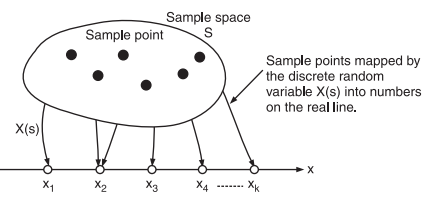# Define Random Variables

## Random variable (RV)

The term Random Variable is used to signify a rule by which a real number is assigned to each possible outcome of an experiment.

If ‘s’ represents the outcome of the experiment, then the random variable is represented by X(s) or simply ‘X’.

Thus, the random variable is a rule or functional relationship.

X(s) is a function that maps the sample points into real numbers x1, x2,…, so, we have a random variable X which takes on the values x1, x2….

Naturally, the term random variable is misnormer since X(s) is not a variable at all and in no way random being a perfectly defined rule.

For example, the sample space representing the outcomes of throwing a die is a set of six sample points from 1 to 6, Now, if we identify the sample point k with the event that k dots show when the die is thrown then the function X(k) = k is a random variable such that X(k) is equal to the number of dots show when the die is thrown.

### Types of Random Variables

The random variables are of following two types:

(i) Discrete random variables

(ii) Continuous random variables

### Discrete Random Variables

As we have already stated, a random variable associated with an experiment is a rule or relationship X(s) that assigns a real number X to every sample point ‘s’.

If the sample space ‘S’ contains a countable number of sample points then X(s) will be a discrete random variable as shown in fig.1.Fig.1 : A discrete random variable

A discrete random variable will thus have a countable number of distinct values. We take the example of an experiment of tossing a coin. The sample space for this experiment is

S = {H, T}

Thus, the sample space consists of a finite number of outcomes. Now, a random variable X(s) will assign two distinct real numbers to these two outcomes of the experiment.

Thus, the random variable also will have two fixed and distinct real numbers. Hence, this random variable X(s) will be a discrete random variable.

### Continuous Random Variable

A continuous random variable is not restricted to a finite number of distinct values.Instead, it can have any value within a certain range.

Thus, the continuous random variable has an ‘uncountable’ number of possible values.

Such a random variable is defined for systems which generate an infinite number of outputs (outcomes) within a finite period of time.